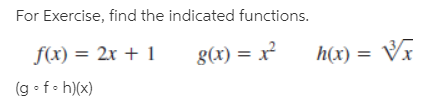# For Exercise, find the indicated functions. f(x) = 2x + 1 (g • f• h)(x) g(x) = x² h(x) = Vx

Questionhelp_outlineImage TranscriptioncloseFor Exercise, find the indicated functions. f(x) = 2x + 1 (g • f• h)(x) g(x) = x² h(x) = Vx fullscreen